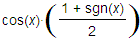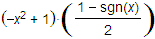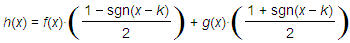﻿ Windows > Calculator > How to Use Signum to Construct a Piecewise Function

# How to Use Signum to Construct a Piecewise FunctionSometimes you may want to construct a function that behaves one way in part of its domain and differently in another part of its domain. Such functions, called piecewise functions, are very important in interpolation, in fitting curves to data, and in designing and producing shapes and surfaces in applications such as automobile design. The signum function makes it possible to do this in Sketchpad.

The signum function is useful any time you want a calculation which makes some sort of decision — which performs a different calculation when some value changes.

For instance, you could construct a function whose plot is in the shape of a cosine wave when x > 0, but is parabolic when x < 0. Here’s how to do this with the signum function:

 1.Choose 2.Enter the cosine-wave part of the function, as shown at right. When x > 0, the signum function returns +1, and the value of the multiplier is 1. But when x < 0, the signum function returns –1, and the value of the multiplier is 0. This first part of the function will be a cosine wave to the right of the origin, but will always be zero to the left of the origin.3.Add the parabola part of the function as shown at right. When x > 0, the multiplier will be 0, and when x < 0, the multiplier will be 1.Here’s the result when you click OK:The functions in this example were chosen in such a way that the two functions join continuously and smoothly. Can you construct a different piecewise function in which the join is not continuous, or in which it’s continuous but not smooth?

Here’s how to use the signum function to solve the general problem of defining a function h(x) whose value is f(x) for all x < k and is g(x) for all x > k: